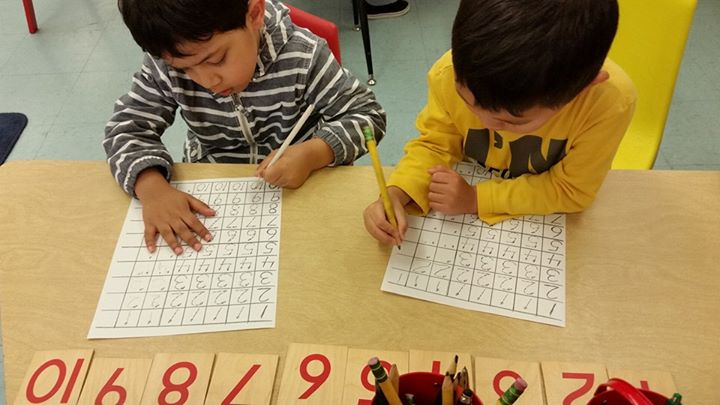The Math area of the Montessori classroom encompasses the use of concrete materials for the recognition of numbers and the recognition of quantity as well. Through these activities, children learn exactly how much a symbolic number stands for (i.e. the number 5 means counting the correct number of objects to make the number 5). Mathematics activities are divided into six categories that include: counting and the decimal system, memory work, concrete abstraction, arithmetic tables and geometry. Children are introduced to more complex mathematical procedures and concepts as they are individually ready. Often times a child will complete a mathematical activity a few times until he feels ready to attempt a concept that is more difficult.